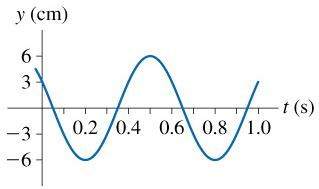# Problem: The figure is a history graph at x=0m of a wave moving to the right at 2m/s.(a) What is the frequency of this wave?(In Hz)(b) What is the wavelength of this wave?

###### FREE Expert Solution

Velocity, frequency, and wavelength relation:

$\overline{){\mathbf{v}}{\mathbf{=}}{\mathbf{f}}{\mathbf{\lambda }}}$

Frequency and periodic time are related as:

$\overline{){\mathbf{f}}{\mathbf{=}}\frac{\mathbf{1}}{\mathbf{T}}}$

1s-1 = 1 Hz

(a)

Periodic time is the time from crest to crest.

99% (84 ratings)###### Problem DetailsThe figure is a history graph at x=0m of a wave moving to the right at 2m/s.

(a) What is the frequency of this wave?(In Hz)

(b) What is the wavelength of this wave?

Frequently Asked Questions

What scientific concept do you need to know in order to solve this problem?

Our tutors have indicated that to solve this problem you will need to apply the Waves on a String concept. You can view video lessons to learn Waves on a String. Or if you need more Waves on a String practice, you can also practice Waves on a String practice problems.

What professor is this problem relevant for?

Based on our data, we think this problem is relevant for Professor Edwards-Bruner's class at TXSTATE.